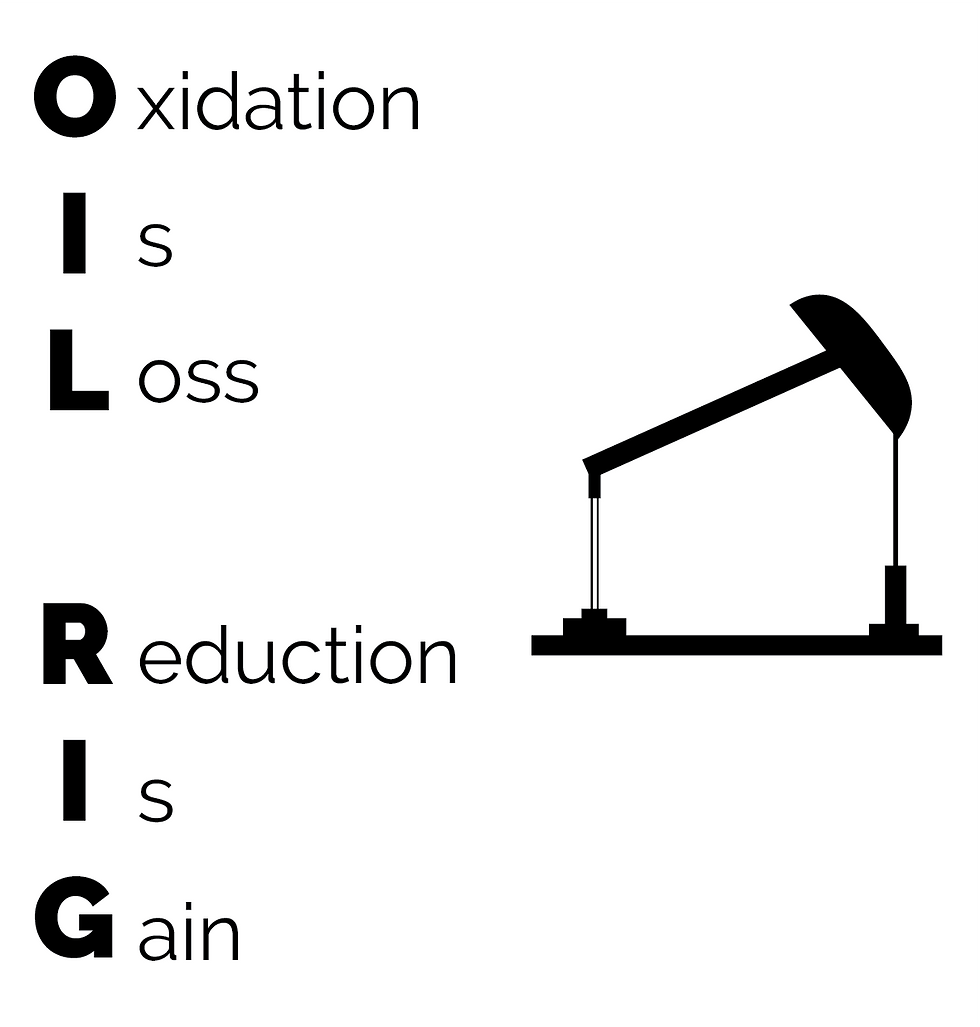cum
top of page
Search

# CSEC Chemistry: Types of Chemical Reactions

While studying chemistry, you will come across several types reactions, and it is often necessary to predict the products of a certain reaction or simply be able to identify any of these types of reactions.

## Direct Combination/Synthesis Reactions

This type of reaction is where two or more substances combine to form a single new substance (product). This is represented by the general equation:

A + B → AB

For example:

Metals react with oxygen to produce the metal oxide (either when heated or at room temperature):

metal + oxygen → metal oxide

2Zn(s) + O2(g) → 2ZnO(s)

When a non metal is heated in oxygen or air, the non-metal oxide is produced:

non-metal + oxygen → non-metal oxide

heat

S(s) + O2(g) → SO2(g)

Some non-metals can react with metals to form a salt:

metal + non-metal → salt

2Na(s) + Cl2(g) → 2NaCl(s)

## Decomposition Reactions

In decomposition reactions, one compound breaks down into two or more simpler substances. This usually requires conditions such as light, heat or electricity. The general form of the equation is this:

AB → A + B

If a compound decomposes in the presence in heat, it is known as thermal decomposition. The products of a decomposition reaction can be elements or compounds.

For example:

Metal hydroxides decompose on heating to yield metal oxides and water.

metal hydroxide → metal oxide + water

heat

2NaOH → Na2O + H2O

Water splits into hydrogen gas and oxygen gas when an electric current is run through it.

2H2O → 2H2 + O2

electrical

current

## Substitution/Displacement Reactions

In a substitution or single displacement reaction, one element replaces a similar one in another compound. This can be shown in the general equation:

A + BX → AX + B

In this case, A is a metal replacing metal B in a compound. However, if a non-metal is replacing another, it looks like this:

Y + XZ → XY + Z

There are three basic types of single displacement reactions:

1) a more reactive metal displaces a less reactive metal from an aqueous solution of its salt:

Zn(s) + CuSO4(aq) → ZnSO4(aq) + Cu(s)

Zinc is more reactive than Copper, so it replaces Copper in its salt. Think of it as both of the metals being magnets, but Zinc is stronger than Copper. So, zinc will attract the sulphate ion more strongly. This really occurs because Zinc is easier to ionize than copper.

2) a reactive metal displaces hydrogen from an acid or water:

Zn(s) + 2HCl(aq) → ZnCl2(aq) + H2(g)

2Na(s) + H2O(l) → 2NaOH(aq) + H2(g)

3) a more reactive non-metal displaces a less reactive non-metal from an aqueous solution of its salt.

F2(g) + 2NaCl(aq) → 2NaF(aq) + Cl2(g)

Fluorine is the most electronegative element on the periodic table, so it will displace any other non-metal from its salt.## Ionic Precipitation/Double Displacement Reactions

Here, two soluble ionic compounds react to form one soluble compound another insoluble precipitate. (In order for a reaction to occur, one of the products must be either an insoluble precipitate, a gas, or a molecular compound like water). The positive and negative ions of both ionic compounds switch places. This can be shown with the general equation:

AB + CD → AD + CB

A and C are positively charged ions (cations) while B and D are negatively charged ions (anions).

For example:

AgNO3(aq) + NaCl(aq) → AgCl(s) + NaNO3(aq)

## Combustion Reactions

These reactions occur when a substance reacts with oxygen gas to release energy as light or heat. Oxygen must always be a reactant.

Most commonly, combustion reactions involve the reaction of an organic compound like a hydrocarbon with oxygen to form carbon dioxide and water.

This can be shown in the general equation:

CₐHₑOₒ + O₂ → CO₂ + H₂O

(a, e and o are arbitrary values)

For example:

C3H8(g) + 5O2(g) → 3CO2(g) + 4H2O(g)

## Neutralization Reactions

Neutralization reaction occur between an acid (or acidic oxide) and a base. The products of a neutralization reaction include water and a salt- but heat is also produced (neutralization reactions are exothermic).

For example:

HCl(aq) + KOH(aq) → H2O(l) + KCl(aq)

This is technically a double displacement reaction.

## Reversible Reactions

Up to this point, we have only discussed reactions which occur unidirectionally, or in one direction from products on the left to reactants on the right. Reversible reactions can occur bidirectionally, or from reactants to products and products to reactions.

A useful example of a reversible reaction is the reaction between nitrogen and hydrogen:

N2(g) + 3H2(g) 2NH3(g)

This reaction (and similar reversible reactions) will proceed in one direction originally. As the amount of ammonia increases, some of it dissociates and the backward reaction begins as well. At some point, the rate of forward and backward reaction will become equal- at which point the reaction vessel would be considered to be in dynamic equilibrium.

## Redox Reactions

These are reactions where reduction and oxidation occur simultaneously.

Reduction is the gain of electrons.

Oxidation is the loss of electrons.

Here's a good acronym to remember this with:We will discuss more on redox reactions in another post.